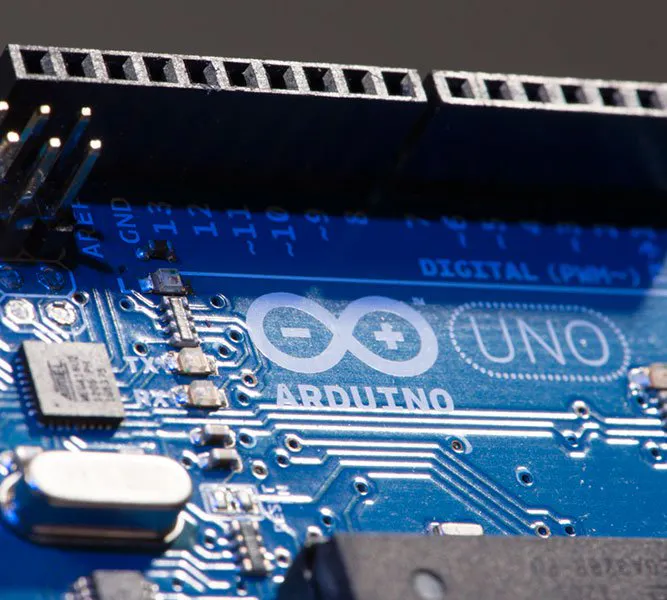# LED Cube 7x7x7

How to make a 7x7x7 LED cube with Arduino.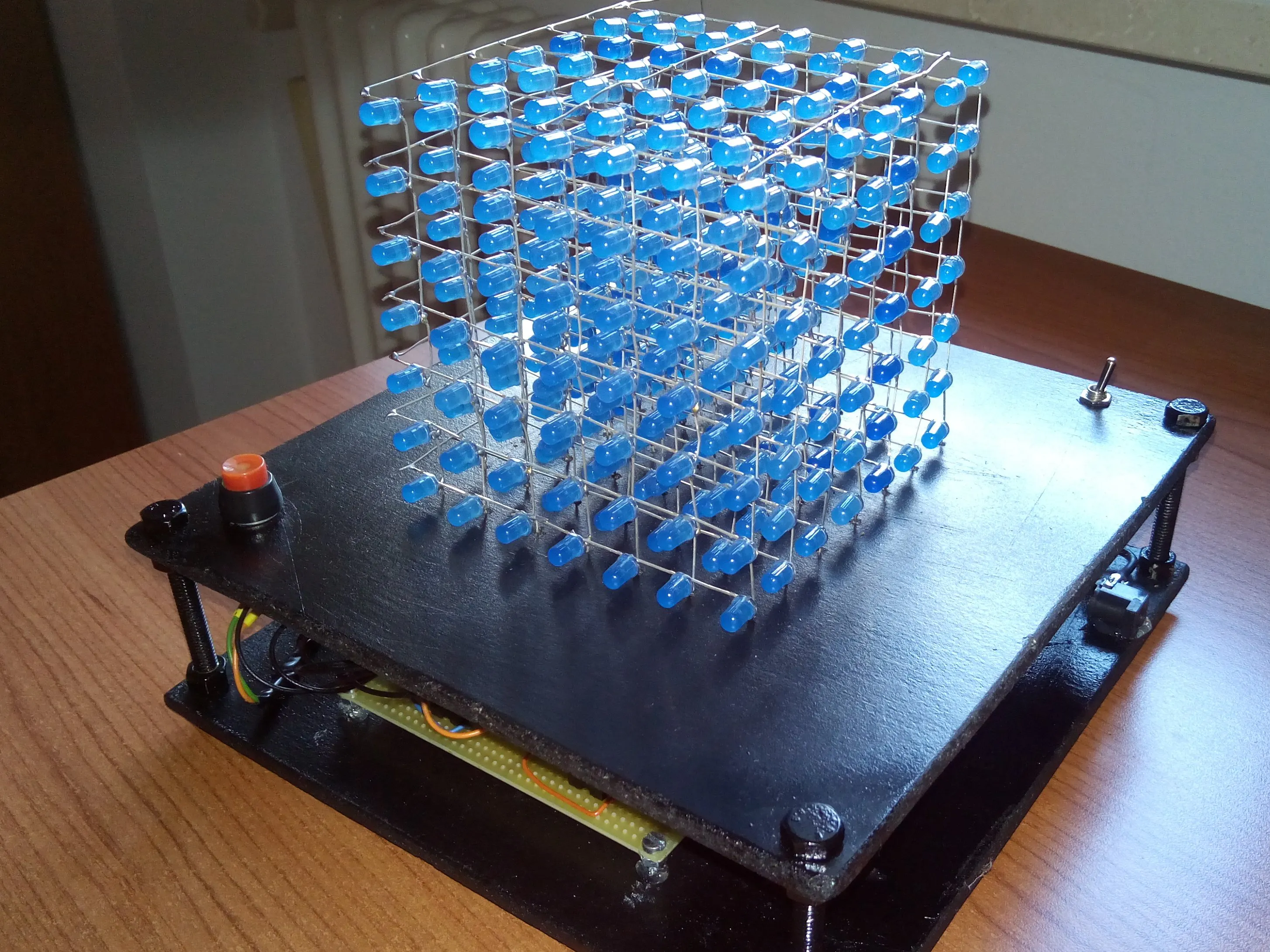## Things used in this project

### Hardware componentsArduino UNO & Genuino UNO
×1
 74hc574
×7
 74hc138
×1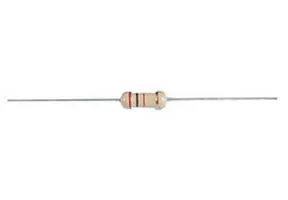Resistor 220 ohm
×49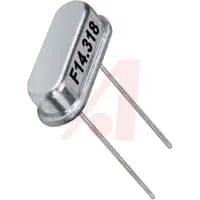16 MHz Crystal
×1

### Hand tools and fabrication machinesSoldering iron (generic)

## Schematics

### control circuit's layout

I use the image for components arrangement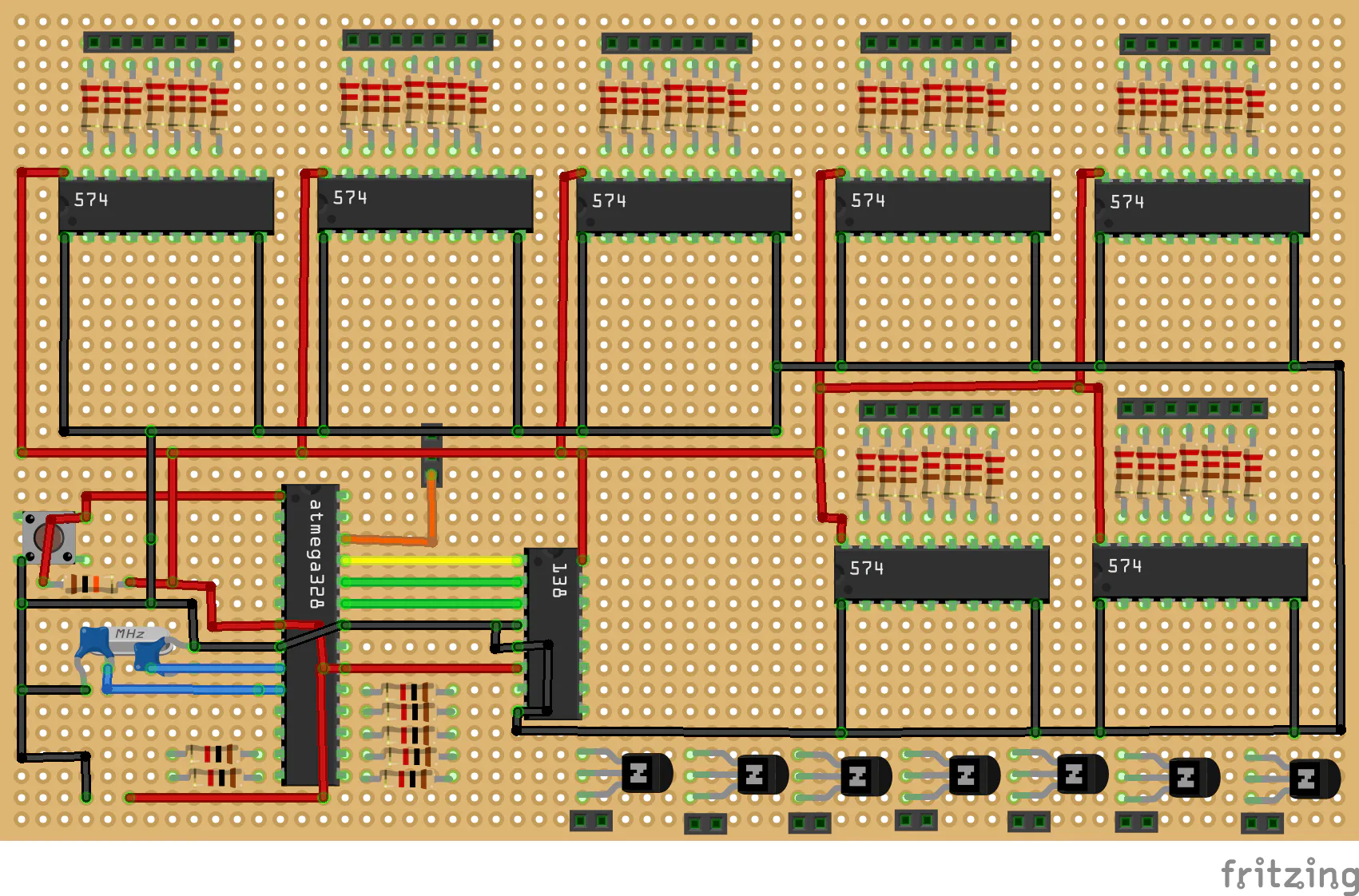## Code

### Animation

Arduino
```//This code represent the easiest animation for LED CUBE

int temp = 50, cont = 0;

//This function loads all the cube
//layer 1
digitalWrite(A2, LOW);
digitalWrite(A1, LOW);
digitalWrite(A0, LOW);
delay(1);

//layer 2
digitalWrite(A2, HIGH);
digitalWrite(A1, LOW);
digitalWrite(A0, LOW);
delay(1);

//layer 3
digitalWrite(A2, LOW);
digitalWrite(A1, HIGH);
digitalWrite(A0, LOW);
delay(1);

//layer 4
digitalWrite(A2, HIGH);
digitalWrite(A1, HIGH);
digitalWrite(A0, LOW);
delay(1);

//layer 5
digitalWrite(A2, LOW);
digitalWrite(A1, LOW);
digitalWrite(A0, HIGH);
delay(1);

//layer 6
digitalWrite(A2, HIGH);
digitalWrite(A1, LOW);
digitalWrite(A0, HIGH);
delay(1);

//layer 7
digitalWrite(A2, LOW);
digitalWrite(A1, HIGH);
digitalWrite(A0, HIGH);
delay(1);

//layer 8
digitalWrite(A2, HIGH);
digitalWrite(A1, HIGH);
digitalWrite(A0, HIGH);
delay(1);

}

//This function actives each layer one time and you can decide the time that the layer turns on
void scrolling(int t) {
PORTB = 0x00;
digitalWrite(7, HIGH);
delay(t);
digitalWrite(7, LOW);

digitalWrite(8, HIGH);
delay(t);
digitalWrite(8, LOW);

digitalWrite(9, HIGH);
delay(t);
digitalWrite(9, LOW);

digitalWrite(10, HIGH);
delay(t);
digitalWrite(10, LOW);

digitalWrite(11, HIGH);
delay(t);
digitalWrite(11, LOW);

digitalWrite(12, HIGH);
delay(t);
digitalWrite(12, LOW);

digitalWrite(13, HIGH);
delay(t);
digitalWrite(13, LOW);

}

//This animation turns on one layer per time, and it increases the speed of it, so finally you see all cube switched on
void one(void) {
PORTD = 0xff;
while (1) {
scrolling(temp);

temp = temp - 2;

if (temp <= 0) {
temp = 1;
cont++;
if (cont == 1000) {
cont = 0;
temp = 100;
}
}

}
}

void setup() {
DDRD = 0xff;
DDRB = 0xff;
DDRC = 0xff;

}

void loop() {
one();
}
```

## Credits

### Marcazzan_M

4 projects • 46 followers
I'm an Italian student, I'm studying electronic engineering. Sufficiently advanced technology is indistinguishable from magic.